# Math Block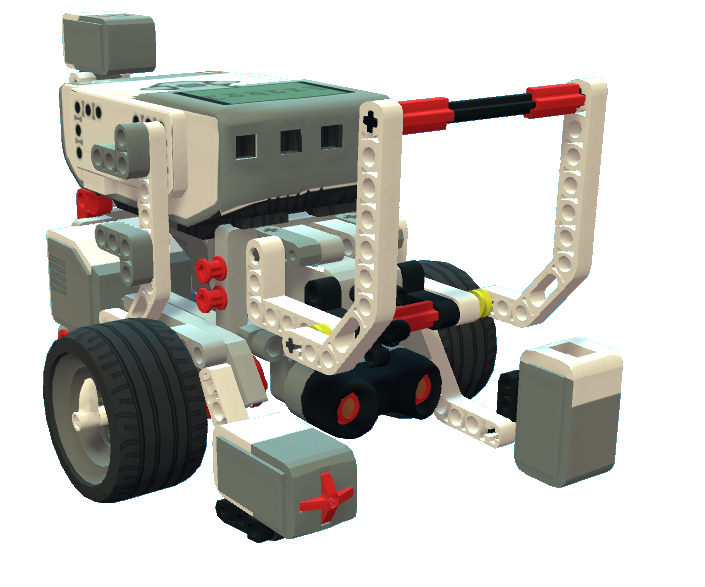This block works for both EV3, Zappy, and Ruby!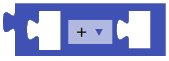Numbers are all around us, and the numerical operators allow you to perform mathematical operations between two numbers. These numbers can be constants or variables.

Insert two numbers (or variables) and use the block's drop-down menu to choose between the various operations.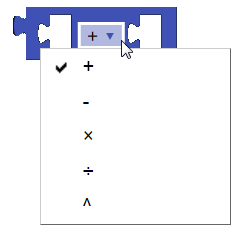Example Program

In this program, the Set Variable block causes the power of the Drive block to increase by 1 each second (for each iteration of the loop).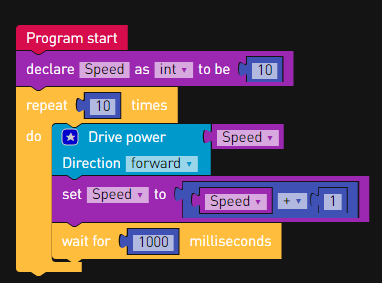Configuration

 Description Compatible Blocks Range Performs a mathematical operation between two numbers.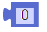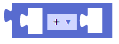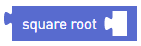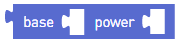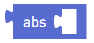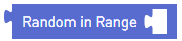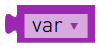-∞ to ∞